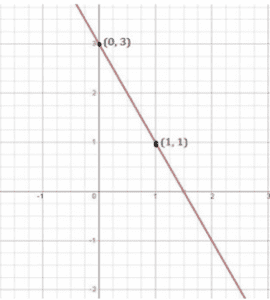# Draw the graph of the following linear equations in two variables: 3 = 2x + y

To draw the graph 3=2x+y, we have to find the values which x and y can have, satisfying the equation.

We can check that when, x = 0, y =3,

When x = 0,

3 = 2x+y

3 = 2×0+y

3 = 0+y

y = 3

When x = 1,

3= 2x+y

3 = 2×1+y

3 = 2+y

y = 3–2

y = 1

are solutions of the given equation. So, we can use the following table to draw the graph:

 x y 0 3 1 1

The points to be plotted are (0, 3) and (1, 1)